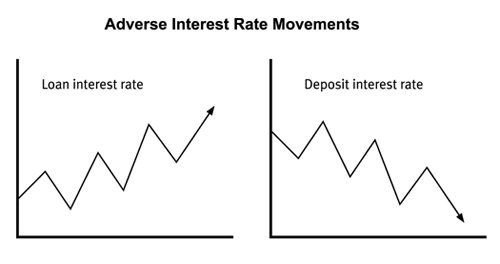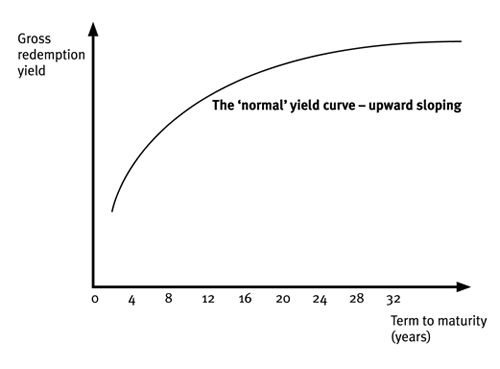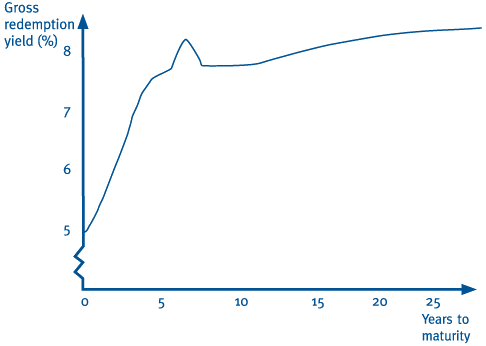ACCAPEDIA

Kaplan Wiki > ACCAPEDIA > Wiki Pages > Interest Rate Risk Management

Interest Rate Risk ManagementFavorite|Discussions
| |History
|Quick Launch View All Site ContentInterest rate risk management

Back up a level
 Contents [Hide]

Interest rate risk management

Financial managers face risk arising from changes in interest rates, i.e. a lack of certainty about the amounts or timings of cash payments and receipts.

Many companies borrow, and if they do they have to choose between borrowing at a fixed rate of interest (usually by issuing bonds) or borrow at a floating (variable) rate (possibly through bank loans).There is some risk in deciding the balance or mix between floating rate and fixed rate debt. Too much fixed-rate debt creates an exposure to falling long-term interest rates and too much floating-rate debt creates an exposure to a rise in short-term interest rates.

In addition, companies face the risk that interest rates might change between the point when the company identifies the need to borrow or invest and the actual date when they enter into the transaction.

Managers are normally risk-averse, so they will look for techniques to manage and reduce these risks.

Interest rate risk exposure

Interest rate risk

Interest rate risk refers to the risk of an adverse movement in interest rates and thus a reduction in the company's net cash flow.Compared to currency exchange rates, interest rates do not change continually:

• currency exchange rates change throughout the day
• interest rates can be stable for much longer periods

but changes in interest rates can be substantial.

It is the duty of the corporate treasurer to reduce (hedge) the company's exposure to the interest rate risk.

Gap exposure

The degree to which a firm is exposed to interest rate risk can be identified through gap analysis. This uses the principle of grouping together assets and liabilities that are affected by interest rate changes according to their maturity dates. Two different types of gap may occur:

• A negative gap occurs when interest-sensitive liabilities maturing at a certain time are greater than interest-sensitive assets maturing at the same time. This results in a net exposure if interest rates rise by the time of maturity;
• A positive gap occurs is the amount of interest-sensitive assets maturing in a certain period exceeds the amount of interest-sensitive liabilities maturing at the same time. In this situation the firm will lose out if interest rates fall by maturity.

Practical examples of interest rate risk

The following are all practical examples of interest rate risk:

A company might borrow at a variable rate of interest, with interest payable every six months and the amount of the interest charged each time varying according to whether short-term interest rates have risen or fallen since the previous payment.

Some companies borrow by issuing bonds. If a company foresees a future requirement to borrow by issuing bonds, it will have an exposure to interest rate risk until the bonds are eventually issued.

Some companies also budget to receive large amounts of cash, and so budget large temporary cash surpluses that can be invested short-term. Income from those temporary investments will depend on what the interest rate happens to be when the money is available for depositing.

Some investments earn interest at a variable rate of interest (e.g.money in bank deposit accounts) and some short-term investments go up or down in value with changes in interest rates (for example, Treasury bills and other bills).

Some companies hold investments in marketable bonds, either government bonds or corporate bonds. These change in value with movements in long-term interest rates.

Interest rate risk can be significant. For example, suppose that a company wants to borrow \$10 million for one year, but does not need the money for another three weeks. It would be expensive to borrow money before it is needed, because there will be an interest cost. On the other hand, a rise in interest rates in the time before the money is actually borrowed could also add to interest costs. For example, a rise of just 0.25% (25 basis points) in the interest rate on a one-year loan of \$10 million would cost an extra \$25,000 in interest over the course of a year.

Hedging interest rate risk

Most tools for hedging interest rate risk can be grouped into two categories:

Tools to fix the rate

Forward rate agreements or FRAs

Interest rate futures

Tools to give the option to fix the rate

Interest rate guarantees or IRGs

Options on interest rate futures

Interest rate collars

In addition to these we have interest rate swaps.

Further detail - why interest rates fluctuate

The yield curve

The term structure of interest rates refers to the way in which the yield (return) of a debt security or bond varies according to the term of the security, i.e. to the length of time before the borrowing will be repaid.

The yield curve is an analysis of the relationship between the yields on debt with different periods to maturity.

A yield curve can have any shape, and can fluctuate up and down for different maturities.

There are three main types of yield curve shapes: normal, inverted and flat (or humped):

• normal yield curve - longer maturity bonds have a higher yield compared with shorter-term bonds due to the risks associated with time
• inverted yield curve - the shorter-term yields are higher than the longer-term yields, which can be a sign of upcoming recession
• flat (or humped) yield curve - the shorter- and longer-term yields are very close to each other, which is also a predictor of an economic transition.

The slope of the yield curve is also seen as important: the greater the slope, the greater the gap between short- and long-term rates.In reality the curve often has a wiggle:What explains the shape of the yield curve?

The shape of the yield curve at any point in time is the result of the three following theories acting together:

• liquidity preference theory
• expectations theory
• market segmentation theory.

Liquidity preference theory

Investors have a natural preference for holding cash rather than other investments, even low-risk ones such as government securities.They therefore need to be compensated with a higher yield for being deprived of their cash for a longer period of time. The normal shape of the curve as being upwards sloping can be explained by liquidity preference theory.

Expectations theory

This theory states that the shape of the yield curve varies according to investors' expectations of future interest rates.

A curve that rises steeply from left to right indicates that rates of interest are expected to rise in the future. There is more demand for short-term securities than long-term securities since investors' expectation is that they will be able to secure higher interest rates in the future so there is no point in buying long-term assets now. The price of short-term assets will be bid up, the price of long-term assets will fall, so the yields on short-term and long-term assets will consequently fall and rise.

A falling yield curve (also called an inverted curve, since it represents the opposite of the usual situation) implies that interest rates are expected to fall.

In the early 1990s UK interest rates were high to counteract high inflation. Everybody expected interest rates to fall in the future, which they did. Expectations that interest rates would fall meant it was cheaper to borrow long-term than short-term.

A flat yield curve indicates expectations that interest rates are not expected to change materially in the future.

Market segmentation theory

The market segmentation theory suggests that there are different players in the short-term end of the market and the long-term end of the market. If there is an increased supply in the long-term end of the market because the government needs to borrow more, this may cause the price to f all and the yield to rise and may result in an upward sloping yield curve

As a result of the market segmentation theory, the two ends of the curve may have different shapes, as they are influenced independently by different factors.

• Investors are assumed to be risk averse and to invest in segments of the market that match their liability commitments, e.g.
• banks tend to be active in the short-term end of the market
• pension funds would tend to invest in long-term maturities to match the long-term nature of their liabilities.
• The supply and demand forces in various segments of the market in part influence the shape of the yield curve.

Market segmentation theory explains the 'wiggle' seen in the middle of the curve where the short end of the curve meets the long end - it is a natural disturbance where two different curves are joining and the influence of both the short-term factors and the long-term factors are weakest.

The significance of the yield curve

Financial managers should inspect the current shape of the yield curve when deciding on the term of borrowings or deposits, since the curve encapsulates the market's expectations of future movements in interest rates.

For example, a normal upward sloping yield curve suggests that interest rates will rise in the future. The manager may therefore:

• wish to avoid borrowing long-term on variable rates, since the interest charge may increase considerably over the term of the loan
• choose short-term variable rate borrowing or long-term fixed rate instead.

Expectations of future interest rate movements are monitored closely by the financial markets, and are important for any organisation that intends to borrow heavily or invest heavily in interest-bearing instruments. A company might use a 'forward yield curve' to predict what interest rates might be in the future. For example, if we know the current interest rate on a two-month and a six-month investment, it is possible to work out what the market expects the four-month interest rate to be in two months' time.

A corporate treasurer might analyse a yield curve to decide for how long to borrow. For example, suppose a company wants to borrow \$20million for five years and would prefer to issue bonds at a fixed rate of interest. One option would be to issue bonds with a five-year maturity. Another option might be to borrow short-term for one year,say, in the expectation that interest rates will fall, and then issue a four-year bond. When borrowing large amounts of capital, a small difference in the interest rate can have a significant effect on profit.  So if the yield curve indicates that interest rates are expected to fall then short-term borrowing for a year, followed by a 4-year bond might be the cheapest option.Created at 9/13/2012 10:21 AM  by System Account  (GMT) Greenwich Mean Time : Dublin, Edinburgh, Lisbon, London Last modified at 11/13/2012 3:43 PM  by System Account  (GMT) Greenwich Mean Time : Dublin, Edinburgh, Lisbon, LondonRating :Ratings & Comments  (Click the stars to rate the page)Tags:Recent Discussions

 There are no items to show in this view.ACCAPEDIA - Interest Rate Risk Management